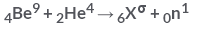NEET  >  Test: Nuclear Energy

# Test: Nuclear Energy

Test Description

## 10 Questions MCQ Test Physics Class 12 | Test: Nuclear Energy

Test: Nuclear Energy for NEET 2023 is part of Physics Class 12 preparation. The Test: Nuclear Energy questions and answers have been prepared according to the NEET exam syllabus.The Test: Nuclear Energy MCQs are made for NEET 2023 Exam. Find important definitions, questions, notes, meanings, examples, exercises, MCQs and online tests for Test: Nuclear Energy below.
Solutions of Test: Nuclear Energy questions in English are available as part of our Physics Class 12 for NEET & Test: Nuclear Energy solutions in Hindi for Physics Class 12 course. Download more important topics, notes, lectures and mock test series for NEET Exam by signing up for free. Attempt Test: Nuclear Energy | 10 questions in 10 minutes | Mock test for NEET preparation | Free important questions MCQ to study Physics Class 12 for NEET Exam | Download free PDF with solutions
 1 Crore+ students have signed up on EduRev. Have you?
Test: Nuclear Energy - Question 1

### Artificial disintegration with alpha particles of which of the following led to the discovery of neutron?

Detailed Solution for Test: Nuclear Energy - Question 1

James Chadwick set up an experiment to test his hypothesis. Chadwick put a piece of beryllium in a vacuum chamber with some polonium. The polonium emitted alpha rays, which struck the beryllium. When struck, the beryllium emitted the mysterious neutral rays.

Test: Nuclear Energy - Question 2

### In a nuclear reactor:

Detailed Solution for Test: Nuclear Energy - Question 2

A moderator is used in nuclear reactors in order to slow down the speed of neutrons.
In nuclear engineering, a neutron moderator is a medium that reduces the speed of fast neutrons, thereby turning them into thermal neutrons capable of sustaining a nuclear chain reaction involving uranium-235 or a similar fissile nuclide.
Clearly, an obvious way to make a reactor work, and to make use of this characteristic of the 235U(n,f) cross-section, is to slow down the fast, fission neutrons. This can be accomplished, for example, when the neutrons collide with nuclei and scatter in some substance (a moderator).

Test: Nuclear Energy - Question 3

### What is the binding energy of the hydrogen nucleus?​

Detailed Solution for Test: Nuclear Energy - Question 3

Nucleus of hydrogen has only one proton. Hence its binding energy will be zero. The nuclear binding energy is the energy it takes to separate all the nucleons in a nucleus from each other. Since there is only the one nucleon, it's already separated from any other nucleons.

Test: Nuclear Energy - Question 4

The fusion of hydrogen into helium is more likely to take place:

Detailed Solution for Test: Nuclear Energy - Question 4

The fusion of hydrogen into helium is more likely to take place at high temperature and high pressure, like the sun.

Test: Nuclear Energy - Question 5

Given M = mass of the nucleus, A = atomic mass. What is packing fraction?

Detailed Solution for Test: Nuclear Energy - Question 5

Packing fraction: - It tells about the stability of a nucleus.
Packing fraction=isotonic mass=molecular mass (atomic mass)/atomic mass
p.f.=M-A/A

Test: Nuclear Energy - Question 6

Why does the fusion occur at high temperature?

Detailed Solution for Test: Nuclear Energy - Question 6

Fusion reactions takes place at temperature about 107K. It requires this high temperature so that nucleus are moving at very high speed, so that they have high kinetic energy and can overcome the repulsion between nuclei and come together.

Test: Nuclear Energy - Question 7

What amount of energy is released in the fission of 95U235 ?​

Detailed Solution for Test: Nuclear Energy - Question 7

The fission process represented by the equation, 92​U235+0​n156​Ba144+36​Kr89+30​n1
Masses of reactants =234.39+1.01=235.4amu
Masses of products =143.28+88.89+3(1.01) =235.2amu
Energy released = mass difference =235.4−235.2=0.2amu=0.2×931∼200MeV

Test: Nuclear Energy - Question 8

In the following reactionWhat is following value of a?​

Detailed Solution for Test: Nuclear Energy - Question 8

The sum of the atomic no. and atomic mass no. on the reactant and product should be equal .
therefore 9+4 = a+1
a = 12

Test: Nuclear Energy - Question 9

Which of the following statements is true for nuclear forces?

Detailed Solution for Test: Nuclear Energy - Question 9

Nuclear forces are short range forces. This is the only correct answer. Others are wrong. They are the strongest forces in nature and do not obey inverse square law.

Test: Nuclear Energy - Question 10

A fusion bomb involves:​

Detailed Solution for Test: Nuclear Energy - Question 10

Nuclear fusion is the process by which two or more atomic nuclei join together, or “fuse,” to form a single heavier nucleus. During this process, matter is not conserved because some of the mass of the fusing nuclei is converted to energy, which is released.

## Physics Class 12

157 videos|452 docs|213 tests
 Use Code STAYHOME200 and get INR 200 additional OFF Use Coupon Code
Information about Test: Nuclear Energy Page
In this test you can find the Exam questions for Test: Nuclear Energy solved & explained in the simplest way possible. Besides giving Questions and answers for Test: Nuclear Energy, EduRev gives you an ample number of Online tests for practice

## Physics Class 12

157 videos|452 docs|213 tests

### How to Prepare for NEET

Read our guide to prepare for NEET which is created by Toppers & the best Teachers